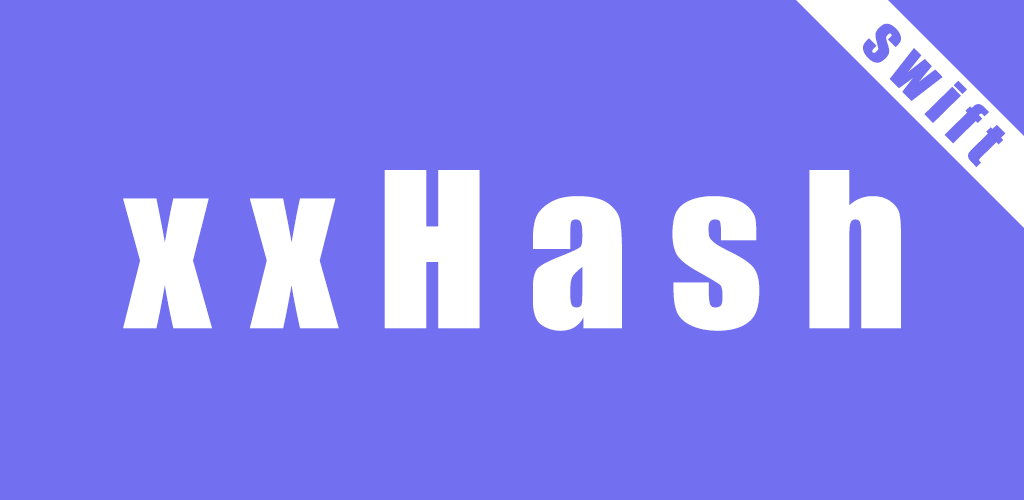Latest 1.0.9 https://github.com/daisuke-t-jp/xxHash-Swift MIT ios 10.0, osx 10.12, tvos 12.0, requires ARC daisuke-t-jpIntroduction

xxHash framework in Swift.
A framework includes xxHash 32/64 bit functions.

Original xxHash algorithm created by Yann Collet.

Documentation

Requirements

• Platforms
• iOS 10.0+
• macOS 10.12+
• tvOS 12.0+
• Linux
• Swift 4.2+

Installation

Carthage

github "daisuke-t-jp/xxHash-Swift"

CocoaPods

use_frameworks!

target 'target' do
pod 'xxHash-Swift'
end

Swift Package Manager

import PackageDescription

let package = Package(
name: "YOUR_PACKAGE_NAME",
targets: [],
dependencies: [
.package(url: "https://github.com/daisuke-t-jp/xxHash-Swift.git", from: "1.0.0")
]
targets: [
.target(
name: "YOUR_TARGET_NAME",
dependencies: ["xxHash-Swift"]),
]
)

Usage

Import framework

import xxHash_Swift

Generate digest(One-shot)

32bit Version

let digest = xxHash32.digest("123456789ABCDEF")
// digest -> 0x576e3cf9

// Using seed.
let digest = xxHash32.digest("123456789ABCDEF", seed: 0x7fffffff)
// digest -> 0xa7f06f9d

64bit Version

let digest = xxHash64.digest("123456789ABCDEF")
// digest -> 0xa66df83f00e9202d

// Using seed.
let digest = xxHash64.digest("123456789ABCDEF", seed: 0x000000007fffffff)
// digest -> 0xe8d84202a16e482f

Generate digest(Streaming)

32bit Version

// Create xxHash instance
let xxh = xxHash32() // if using seed, e.g. "xxHash32(0x7fffffff)"

// Get data from file
let bundle = Bundle(for: type(of: self))
let path = bundle.path(forResource: "alice29", ofType: "txt")!
let data = NSData(contentsOfFile: path)! as Data

let bufSize = 1024
var index = 0

repeat {
var lastIndex = index + bufSize
if lastIndex > data.count {
lastIndex = index + data.count - index
}

let data2 = data[index..<lastIndex]
xxh.update(data2) // xxHash update

index += data2.count
if index >= data.count {
break
}
} while(true)

let digest = xxh.digest()
// digest -> 0xafc8e0c2

64bit Version

// Create xxHash instance
let xxh = xxHash64() // if using seed, e.g. "xxHash64(0x0000007fffffff)"

// Get data from file
let bundle = Bundle(for: type(of: self))
let path = bundle.path(forResource: "alice29", ofType: "txt")!
let data = NSData(contentsOfFile: path)! as Data

let bufSize = 1024
var index = 0

repeat {
var lastIndex = index + bufSize
if lastIndex > data.count {
lastIndex = index + data.count - index
}

let data2 = data[index..<lastIndex]
xxh.update(data2) // xxHash update

index += data2.count
if index >= data.count {
break
}
} while(true)

let digest = xxh.digest()
// digest -> 0x843c2c4ccfbfb749

Latest podspec

{
"name": "xxHash-Swift",
"version": "1.0.9",
"summary": "xxHash framework in Swift.",
"description": "xxHash framework in Swift.nA framework includes xxHash 32/64 bit functions.n nOriginal xxHash algorithm created by Yann Collet.nhttps://cyan4973.github.io/xxHash/",
"homepage": "https://github.com/daisuke-t-jp/xxHash-Swift",
"type": "MIT",
},
"authors": {
"daisuke-t-jp": "[email protected]"
},
"platforms": {
"ios": "10.0",
"osx": "10.12",
"tvos": "12.0"
},
"source": {
"git": "https://github.com/daisuke-t-jp/xxHash-Swift.git",
"tag": "1.0.9"
},
"source_files": "Sources/xxHash/*.{swift}",
"swift_version": "4.2",
"requires_arc": true
}